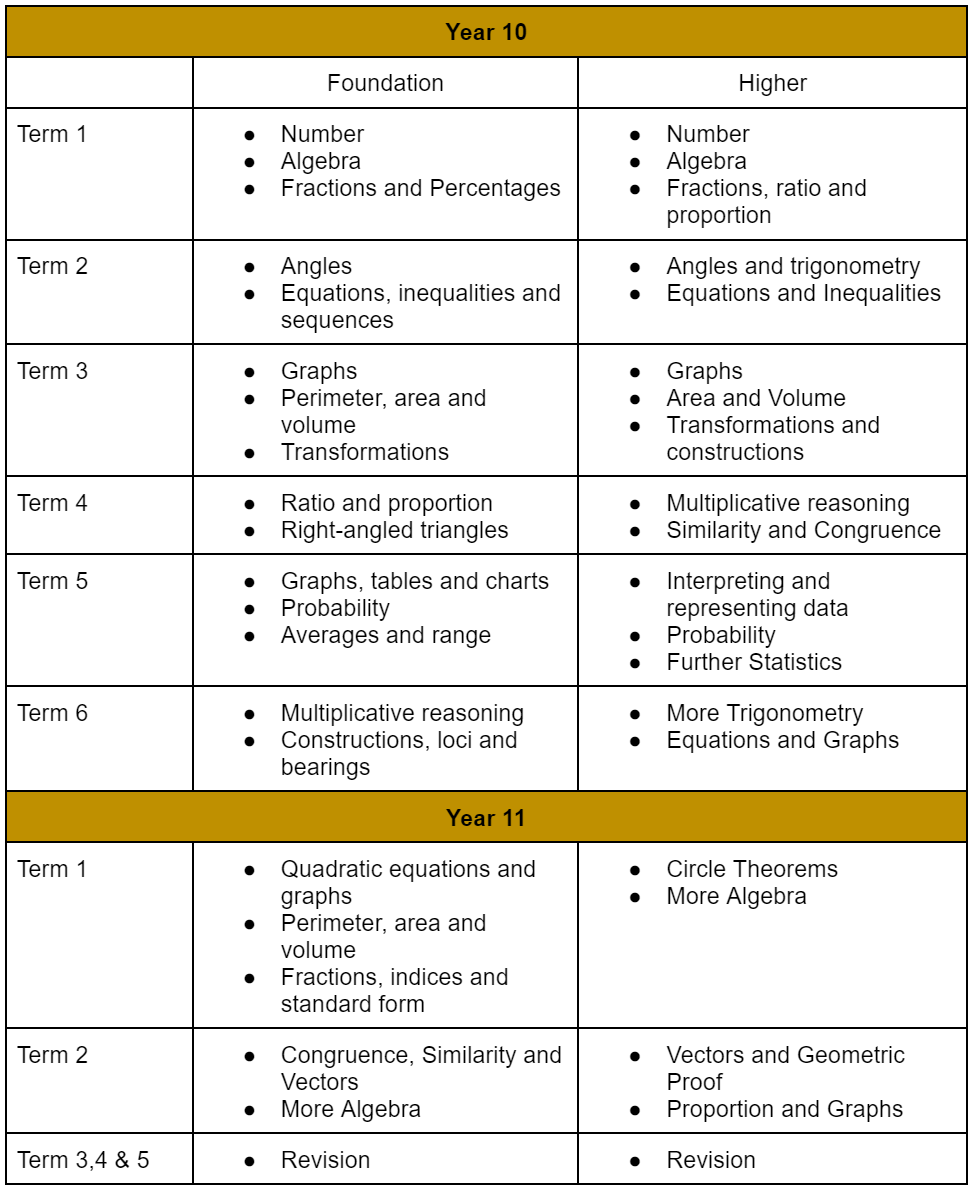## Curriculum# Mathematics

Key Stage 4 Mathematics Curriculum Intent

Our core purpose at Key Stage 4 is to deliver an engaging and challenging curriculum through outstanding teaching and learning. We aim to give them the best opportunities to learn the Key Stage 4 curriculum, to have the best possible outcomes for their GCSE examinations in year 11 and to make them confident and responsible members of the school and wider community. The sequencing of the curriculum enables students to build on their knowledge and skills towards agreed end points.

Mathematics is a creative and highly inter-connected discipline that has been developed over centuries, providing the solution to some of history’s most intriguing problems. It is essential to everyday life, critical to science, technology and engineering, and necessary for financial literacy and most forms of employment. A high-quality mathematics education therefore provides a foundation for understanding the world, the ability to reason mathematically, an appreciation of the beauty and power of mathematics, and a sense of enjoyment and curiosity about the subject. The mathematics curriculum at Meopham School aims to develop character by ensuring that all pupils:

Through the mathematics content, pupils should be taught to:

Develop fluency

• consolidate their numerical and mathematical capability from key stage 3 and extend their understanding of the number system to include powers, roots {and fractional indices}

• select and use appropriate calculation strategies to solve increasingly complex problems, including exact calculations involving multiples of π {and surds}, use of standard form and application and interpretation of limits of accuracy

• consolidate their algebraic capability from key stage 3 and extend their understanding of algebraic simplification and manipulation to include quadratic expressions, {and expressions involving surds and algebraic fractions}

• extend fluency with expressions and equations from key stage 3, to include quadratic equations, simultaneous equations and inequalities

• move freely between different numerical, algebraic, graphical and diagrammatic representations, including of linear, quadratic, reciprocal, {exponential and trigonometric} functions

• use mathematical language and properties precisely.

Reason mathematically

• extend and formalise their knowledge of ratio and proportion, including trigonometric ratios, in working with measures and geometry, and in working with proportional relations algebraically and graphically

• extend their ability to identify variables and express relations between variables algebraically and graphically

• make and test conjectures about the generalisations that underlie patterns and relationships; look for proofs or counter-examples; begin to use algebra to support and construct arguments {and proofs}

• reason deductively in geometry, number and algebra, including using geometrical constructions

• interpret when the structure of a numerical problem requires additive, multiplicative or proportional reasoning

• explore what can and cannot be inferred in statistical and probabilistic settings, and express their arguments formally

• assess the validity of an argument and the accuracy of a given way of presenting information.

Solve problems

• develop their mathematical knowledge, in part through solving problems and evaluating the outcomes, including multi-step problems

• develop their use of formal mathematical knowledge to interpret and solve problems, including in financial contexts

• make and use connections between different parts of mathematics to solve problems

• model situations mathematically and express the results using a range of formal mathematical representations, reflecting on how their solutions may have been affected by any modelling assumptions

• select appropriate concepts, methods and techniques to apply to unfamiliar and non-routine problems; interpret their solution in the context of the given problem.

In Key Stage 4 pupils will be taught in ability groups within the Foundation or Higher pathway. These sets match their strengths, abilities & interests with regard to mathematics. Each class is assigned a separate, named, programme of study which will follow the class throughout the year with pathways to match pupils’ attainment targets. As a department we feel very strongly about developing pupils' love for the subject and creating a deeper understanding of the processes involved. These lessons will give students a real opportunity to think logically, solve problems, work in groups, develop their oracy skills and challenge their way of thinking. Pupils will be given every opportunity to develop their resourcefulness, resilience, reciprocity and reflectiveness.

Key Stage 4 - Maths Overview